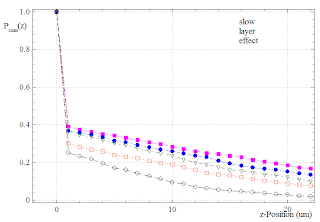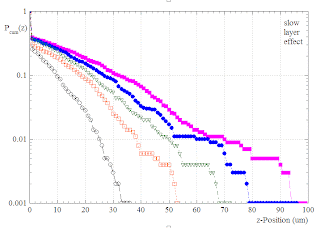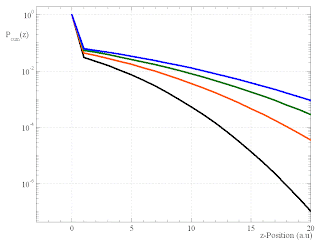## Monday, August 10, 2009

###  Cell-Invasion : Slow Layer Effect

The cell clustering effect, as described in posts  and , is not easy to treat analytically. A much simpler, but somewhat similar model is the "slow layer effect":

The particles (tumor cells) undergo standard diffusion (with rates Rb) in the whole bulk of the material. Only in the z=0-layer the diffusion constant (i.e. the transfer rates for intra layer motion and for steps out of the layer) is reduced to a value Rl smaller than Rb.

Before we go into simulations, a simple one-dimensional consideration shows that the abrupt change of rates at z=0 implies a sudden drop of particle density. We have for the temporal change of density \rho_0 at z=0:

\frac{d}{dt}\rho = -\rho R_l + \rho R_b

If we assume a quasi-steady state, where rho and rho change very slowly with time, we obtain

\frac{\rho}{\rho} = \frac{R_l}{R_b},

and thus rho is much smaller than rho, if R_l is much smaller than R_b.

The following figure shows simulation results for the invasion profiles (cummulative probabilities) in a linear plot. The ratio R_l/R_b was 1/1000. The curves correspond to different times (100,200,...,500 units):One can clearly see the drop of density immediately above the z=0-layer. Note that P_cum acan be interpreted as the fraction of particles that have separated from the boundary layer and invaded the bulk. We might call this quantity the "invaded fraction". It is about 0.4 in the magenta-colored profile above.

A semilog plot of the same results shows that effects of the slow layer range far into the bulk:The shape of P_cum(z) is slightly different from the case of standard diffusion in . The initial "slope" of the curves appears steeper.

Actually, if one removes the single data point at z=0, P_cum(z) can very well be fitted (in the whole bulk z=1...zMax) by shifted Gaussians, using 3 free parameters P_0, z_0 and \sigma:

P_{cum}(z>1) = P_0 \; e^{-(z+z_0)^2/\sigma^2}Basically this means that the curves are approximately factors of exponentials and Gaussians. For not too large z, the exponential factor will dominate. The exponential range should grow with sigma. Therefore, the larger the bulk diffusion constant, the invasion profiles should appear more and more exponential-like. Hence, even the simple "slow layer" model can to some extent account for exponential invasion profiles.

Is it possible to treat this model analytically in 1D ? I guess that in the limit of smaller and smaller width of the z-slices, the effect of the slow layer translates into a simple boundary value condition for the derivative of rho(z). Except this unusual boundary condition, ones has only to solve a standard diffusion Fokker-Planck equation in the bulk.

I tried a very simple numeric solution of the FP equation, using the methods of an old post (Program: Invade1):Note that this is the real particle density P(z), not the cummulative invasion profile. The density jump corresponds to the expected value Rl/Rb. One can also see that the initial slope of the bulk density curves is negative.

Finally, the corresponding cumulative invasion profile: